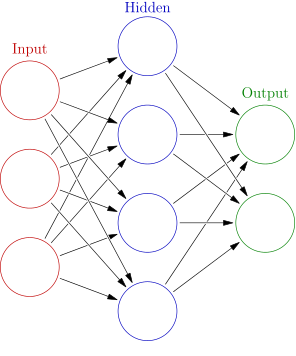In this article you’ll learn about Neural Networks. What is a neural network? The human brain can be seen as a neural network —an interconnected web of neurons .

In Machine Learning, there exist an algorithm known as an Aritifical Neural Network. They mimic biological neural networks. A network always starts with a single unit: the perceptron.

Related course:

## A perceptron

A single perceptron is the basis of a neural network.

A perceptron has:

• one or more inputs
• a bias
• an activation function
• a single outputA perceptron receives inputs. It then multiplies them by some weight. Then they are send into an activation function to produce an output. We also add a bias value, it allows you to shift the activation function.

## Network Layers

A neural network is created by adding layers of perceptrons together: the multi-layer perceptron (MLP) algorithm.

We’ll have several layers:

 Layer Purpose the input layer takes your data the hidden layer any layers between the input and output are called hidden layers output layers the output results

Graphically that looks like this (source: Wikipedia):